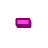Games
Problems
Go Pro!

# Subtraction

Quizzes > Mathematics > Arithmetic > Subtraction

# Featured QuizzesPractice subtraction facts to help improve your ability to solve problems quickly, and without relying on a calculator.Practice your subtraction facts with this simple math quiz from The Problem Site.Practice your subtraction facts with this simple quiz from The Problem Site.Practice your subtraction facts with this simple math quiz from The Problem Site.Practice your subtraction facts with this simple math quiz from The Problem Site.Practice your subtraction facts with this simple math quiz at The Problem Site.Practice your subtraction facts with this simple math quiz at The Problem Site.Practice your subtraction facts with this simple math quiz at The Problem Site.

# Full Directory Listing

Subtraction by 1, Subtraction by 2, Subtraction by 3, Subtraction by 4, Subtraction by 5, Subtraction by 6, Subtraction by 7, Subtraction by 8, Subtraction by 9, Subtraction Facts (All)Like us on Facebook to get updates about new resources